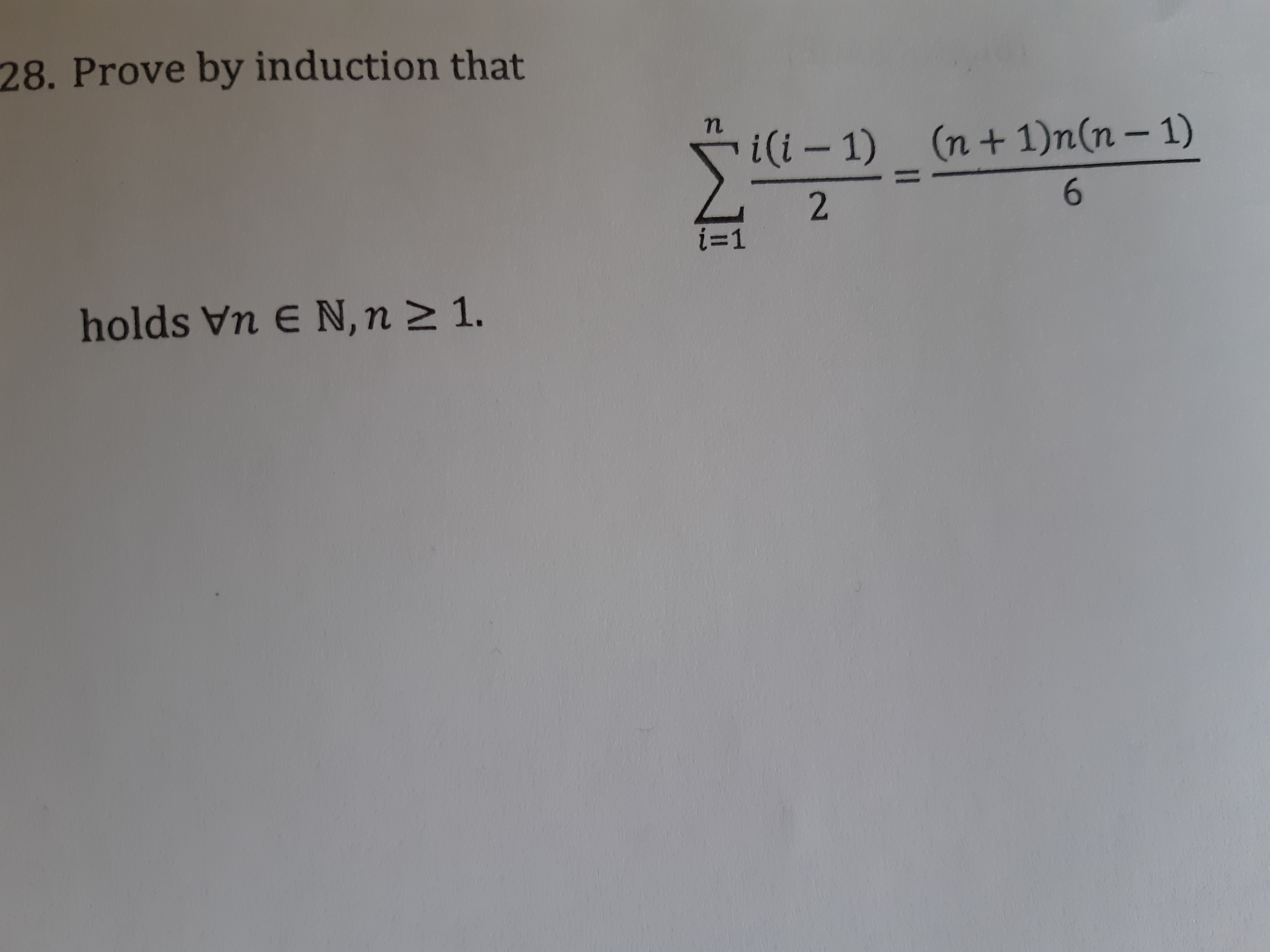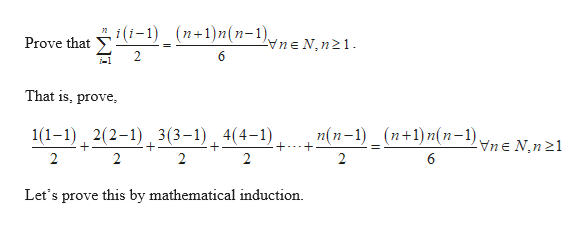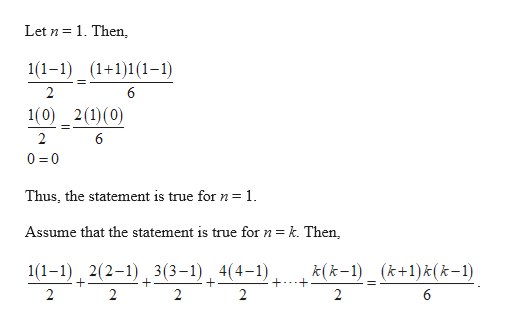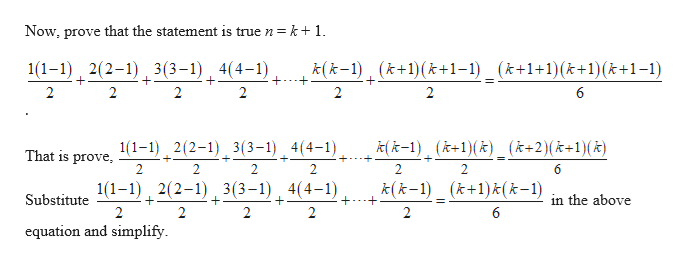# 28. Prove by induction thati(i – 1)(n+1)n(n– 1)i=1holds Vn E N,n 2 1.

Question
75 viewshelp_outlineImage Transcriptionclose28. Prove by induction that i(i – 1) (n+1)n(n– 1) i=1 holds Vn E N,n 2 1. fullscreen
check_circle

star
star
star
star
star
1 Rating
Step 1help_outlineImage Transcriptionclosegi (i-1) (п+1)m(n-1), vne N,n21. Prove that That is, prove, n(n-1) _ (n+1)n(n-1) vne N,n21 1(1-1) , 2(2-1) , 3(3-1), 4(4-1) Let's prove this by mathematical induction. 2. fullscreen
Step 2help_outlineImage TranscriptioncloseLet n = 1. Then, 1(1–1) _ (1+1)1(1-1) 1(0) _ 2(1)(0) 0 = 0 Thus, the statement is true for n=1. Assume that the statement is true for n = k. Then, 1(1–1) , 2(2-1) , 3(3–1), 4(4-1) k(k-1)_ (k+1)k(k-1) 2 2. 2. fullscreen
Step 3help_outlineImage TranscriptioncloseNow, prove that the statement is true n= k+1. 1(1–1) 2(2-1) , 3(3–1) 4(4-1). k(k-1), (k+1)(k+1-1) _ (k+1+1)(k+1)(k+1-1) +-.-+ 2 6 k( k-1), (k+1)(x)_ (k+2)(k+1)(k) 1(1-1) 2(2-1) 3(3-1), 4(4-1) That is prove, 1(1-1), 2(2-1) , 3(3–1) , 4(4-1), k(k-1) _ (k+1)t(k-1) Substitute in the above 2 6 equation and simplify. fullscreen

### Want to see the full answer?

See Solution

#### Want to see this answer and more?

Solutions are written by subject experts who are available 24/7. Questions are typically answered within 1 hour.*

See Solution
*Response times may vary by subject and question.
Tagged in

### Math